27 de julho de 2021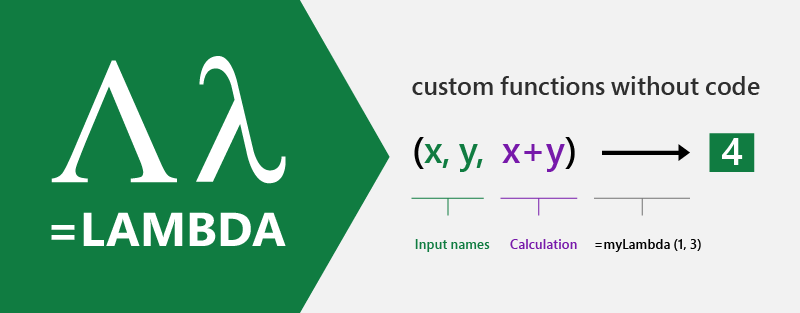## Anunciando funções auxiliares do LAMBDA: Lambdas como argumentos e mais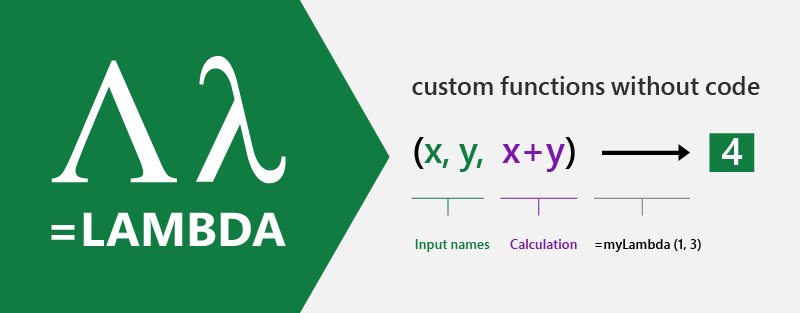Today we are releasing to our Beta customers seven new LAMBDA functions. These functions aid in the authoring of re-usable LAMBDA functions while also serving as stand-alone functions themselves. Additionally, we are sending the LAMBDA function to Current Channel Preview.

LAMBDA as arguments – One exciting addition to Excel’s formula language is that LAMBDA is now exposing the ability to be treated as an accepted value type with the addition of new functions. This is an important concept which has existed across many programming languages and is tantamount to the concept of lambda functions in general.

LAMBDA to Current Channel Preview – With this latest set of updates we are progressing the LAMBDA function to customers who are a part of the Current Channel Preview audience. In addition we are also introducing the ability to define optional arguments.

## Lambdas as Values

Before diving into the new functions and how they work, it’s important to understand the concept of functions as values.

Over the past couple years, we have been teaching Excel how to understand new types of values. Some of the most recent additions have been Data Types (Wolfram, Geography, Stocks, Power BI, and even Power Query can create Data Types), and Dynamic Arrays. Lambdas continue this journey by allowing Excel to understand functions as a value. This was enabled by the introduction of LAMBDAs but not exposed to any functions.

This is exciting, because it means that things which were previously impossible, near-impossible, or arduous can now be achieved by writing a LAMBDA and passing it as a value to a function.

For example, imagine you had a constantly growing list of values and needed to generate a list of booleans which check for values that are both greater than 50 but less than 80.

`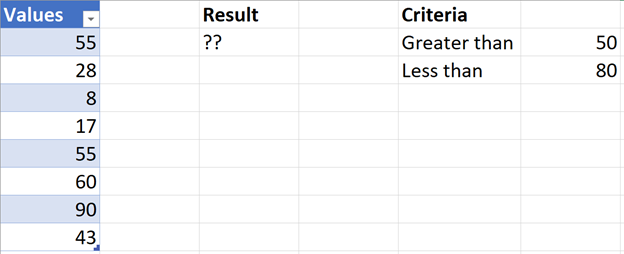`

You could author one function for each value which checks the condition but this is error prone and requires a lot of duplication for something pretty basic. For reference, the duplicated formulas might look like this (with one formula for each value):

`=AND(A2>50, A2=AND(A3>50, A2...`

This is the type of scenario where LAMBDAs can be applied and more specifically, a great example of where to use the new MAP function.

## MAP

With MAP, you can easily author a LAMBDA which applies a formula to every value and returns the result. MAP’s superpower is value transformation.

There are a few new concepts which we will cover shortly, but the formula looks like this:

```=MAP(Table1[Values],LAMBDA(value, AND(value>F2, valueThe result is an array of boolean values, corresponding to the values in the Table: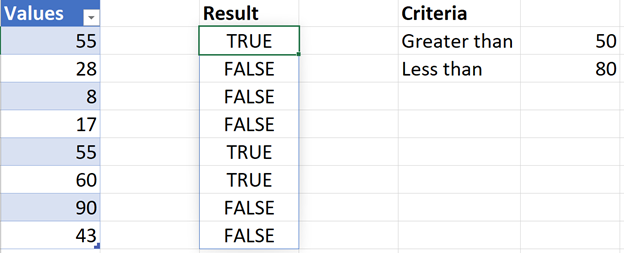With just one formula, you can achieve something pretty powerful! Let’s pause to understand what’s going on.

How the functions work
The new MAP function takes one (or many) array/range references and passes each value from the supplied array/ranges as a parameter to the LAMBDA function (in this case Table1[Values]). The fact that LAMBDA expects parameters from MAP is an important concept to remember as you check out each of the new functions, since it’s different for each one.
In our case we have one array, so the LAMBDA is pretty simple, and expects only one parameter.
Focusing in on the LAMBDA itself, you can see that in this example we chose to call our single parameter “value”, but you can call it by any legal parameter name:

LAMBDA(value, AND(value>F2, valueAnother key concept to understand, with these new functions, is that the power comes from the fact that Excel will do a calculation for each value supplied and then make LAMBDA do the heavy lifting. In this particular case it will return an array of results but there are other functions we are introducing today which will return only one value.

REDUCE
While MAP proves useful for transforming a list of values, let’s say that we wanted to count how many items met the condition.
This is where REDUCE comes in handy and is a perfect opportunity to talk about the second function on our list.
This time, we are going to re-use the same logic as before but wrap the calculation in an IF to do our counting. The formula is rather simple, like before, being that we only need one function call:

=REDUCE(Table1[Values], LAMBDA(accumulator, value,     IF(AND(value>F2, value))
REDUCE does, hopefully, what it sounds like. It reduces a list of values into one value by making use of a LAMBDA.

The major difference with REDUCE is that it makes use of two parameters in its LAMBDA value:

accumulator: The initial value returned by REDUCE and each LAMBDA call.
value: A value from the supplied array

The other thing to note is the first, optional argument, which is the [initial value] for the accumulator. In our case we want it to be 0.
The accumulator is what allows us to write our own custom aggregation-IF function (you can even write PRODUCTIF with REDUCE) and can be seen if you focus on the calculation portion of the LAMBDA:

IF(AND(value>F2, valueThe final result, in my opinion, is elegant and self-contained.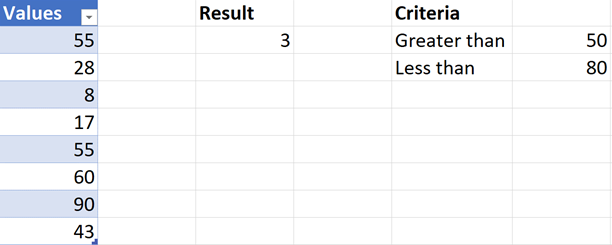BYROW & BYCOL
The next two functions which we are introducing are BYROW and BYCOL. These functions take an array or range and call a lambda with all the data grouped by each row or column and then return an array of single values. Hence the name.
These two functions are great because they allow for calculations which were previously impossible because they would produce arrays of arrays.
For example, lets imagine we had some data which tracked the temperature for every day of a week. We are interested in seeing days where the average temperature for the week is greater than 85 degrees Fahrenheit.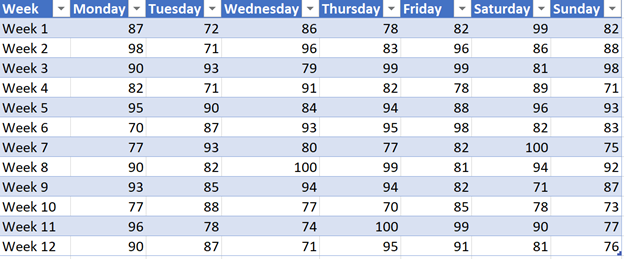Without BYROW, we would need to create a helper column and calculate averages using a bunch of formulas and then likely use filter UI or some other wizardry.
With BYROW, we can author a LAMBDA which meets our constraints and then pass the result to the FILTER function.
This is great because as we add new data week over week, our calculations are set and we don’t have to reauthor our workbook.
The formula looks like this:

=FILTER(Table3, BYROW(Table3, LAMBDA(week, AVERAGE(week)>85)))

And upon execution, we can quickly see which weeks were extra hot!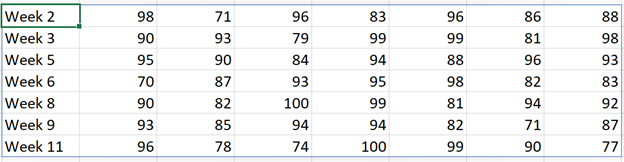Now, let’s suppose, we wanted to see this data in Celsius. We can make a quick update and get results in degrees Celsius by wrapping our call with a MAP and make use of CONVERT.

=MAP(FILTER(Table3, BYROW(Table3, LAMBDA(week, AVERAGE(week)>85))), LAMBDA(value, IF(ISNUMBER(value), CONVERT(value, "F", "C"), value)))

Complete list of functions
While I could create examples for days, I can’t possibly include everything in one post and our documentation does a great job of explaining the specifics of each function, so be sure to check them all out!

Name

Description

Argument

Argument

Argument

LAMBDA_Param(s)

MAP

Returns an array formed by “mapping” each value in the array(s) to a new value by applying a lambda to create a new value.

array1: An array to be mapped.

lambda_or_array:
A LAMBDA which must be the last argument and must have a parameter for each array passed, or another array to be mapped.

[lambda_or_array…]: A LAMBDA which must be the last argument and must have a parameter for each array passed, or another array to be mapped.

array1: A value from array1

[array2…]: A value from an additional array.

REDUCE

Reduces an array to an accumulated value by applying a LAMBDA function to each value and returning the total value in the accumulator.

[initial_value]:
Sets the starting value for the accumulator.

array: An array to be reduced.

lambda: A LAMBDA that is called to reduce the array. The LAMBDA takes two parameters, accumulator and value.

accumulator: The returned value from LAMBDA

value: A value from array.

SCAN

Scans an array by applying a LAMBDA  to each value and returns an array that has each intermediate value.

[initial_value]:
Sets the starting value for the accumulator.

array: An array to be scanned.

lambda: A LAMBDA that is called to scan the array. The LAMBDA takes two parameters, accumulator and value.

accumulator: The returned value from LAMBDA

value: A value from array.

MAKEARRAY

Returns a calculated array of a specified row and column size, by applying a LAMBDA function.

rows: The number of rows in the array. Must be greater than zero.

cols: The number of columns in the array. Must be greater than zero.

lambda: A LAMBDA that is called to create the array. The LAMBDA takes two parameters, row_index and column_index.

row_index: The index of the row.

column_index: The index of the column.

BYROW

Applies a LAMBDA to each row and returns an array of the results.

array:
An array to be separated by row.

[lambda]:
A LAMBDA that takes a row as a single parameter and calculates one result.

value: A value from array.

BYCOL

Applies a LAMBDA to each column and returns an array of the results.

array:
An array to be separated by column.

[lambda]:
A LAMBDA that takes a column as a single parameter and calculates one result.

value: A value from array.

ISOMITTED

Checks whether the value is missing, and returns TRUE or FALSE.

argument: Is the value you want to test, such as a LAMBDA parameter.

LAMBDA Improvements
The last thing to cover is the set of improvements we have made to the LAMBDA feature in general. As aforementioned, we are sending LAMBDA to Current Channel Preview and have added support for Optional Parameters in both Current Channel Preview and Insiders: Beta.

Optional Parameters
LAMBDA now supports optional parameters. To make use of optional parameters, all you need to do is wrap the optional name in “[]”.

For example:
=LAMBDA(param1, [param2], IF(ISOMITTED(param2), param1, param2))

This lambda will return the value of param1 if param2 is omitted and otherwise return the value of param2.

Try it yourself
If you are in our Beta or Current Channel Preview program, you can try out LAMBDAs yourself. We’d love your feedback as we continue to improve on this new capability.
The new LAMBDA functions are available to 50% of the channel today and over the coming weeks we will increase the flight, pending no bugs or other issues.

Note: The LAMBDA function is available to members of the Current Channel Preview program running Windows and Mac builds of Excel. The LAMBDA helper functions are available to members of the Insiders: Beta program running Windows and Mac builds of Excel

Join the Office Insider Program and choose the Beta or Current Channel Preview to get early access to LAMBDA in Excel.  Send us feedback via send a smile, or post in the Excel Tech Community.

Stay connected with us.  Join our Excel Tech Community.  Let us know what you think, and we’d love to see what you build with LAMBDA!

Availability
The new LAMBDA functions are now available to Office Insiders running Beta Channel Version 2108 (Build 14312.20008) or later on Windows, or Version 16.52 (Build 21072100) or later on Mac.

The LAMBDA function is now available to Office Insiders running Current Channel Preview Version 2107 (Build 14228.20154) or later on Windows, or Version 16.51 (Build 21071100) or later on Mac.

To learn more about LAMBDA and the new functions, check out our accompanying post on the Insiders blog which highlights some of the other functions or the help topics below.

LAMBDA Help
New LAMBDA Functions

MAP
REDUCE
SCAN
MAKEARRAY
BYROW
BYCOL
ISOMITTED

```

Artigo originalmente postado em Excel Blog